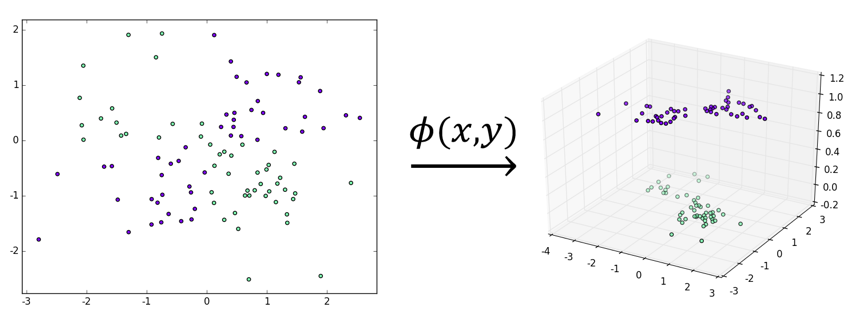# 核技巧简述• 将算法表述成样本点内积的组合（这经常能通过算法的对偶形式实现）
• 设法找到核函数$K\left( x_i,x_j\right)$，它能返回样本点$x_i$$x_j$$\phi$作用后的内积
• $K\left( x_i,x_j\right)$替换$x_i\cdot x_j$、完成低维到高维的映射（同时也完成了从线性算法到非线性算法的转换）

• 对任何平方可积$g$、满足
• 对含任意 N 个样本的数据集$D=\left\{ x_1,...,x_N\right\}$、核矩阵： 是半正定矩阵

Mercer 定理为寻找核函数带来了极大的便利。可以证明如下两族函数都是核函数：

• 多项式核

# 核技巧的应用

1. 输入：训练数据集$D = \{\left( x_{1},y_{1} \right),\ldots,\left( x_{N},y_{N} \right)\}$、迭代次数 M、学习速率$\eta$，其中：
2. 过程

1. 初始化参数：

同时计算核矩阵：

2. $j = 1,\ldots,M$

1. $E = \varnothing$（亦即没有误分类的样本点）则退出循环体
2. 否则，任取$E$中的一个样本点$(x_{i},y_{i})$并利用其下标更新局部参数：
3. 利用$dw$$db$更新预测向量$\hat{y}$其中、$\mathbf{K}_{i\mathbf{\cdot}}$表示$\mathbf{K}$的第 i 行、$\mathbf{1}$表示全为 1 的向量
3. 输出：感知机模型$g\left( x \right) = \text{sign}\left( f\left( x \right) \right) = sign\left( \sum_{i = 1}^{N}{\alpha_{i}y_{i}\left( K\left( x_{i},x \right) + 1 \right)} \right)$

1. 输入：训练数据集$D = \{\left( x_{1},y_{1} \right),\ldots,\left( x_{N},y_{N} \right)\}$、迭代次数 M、容许误差$\epsilon$，其中：
2. 过程

1. 初始化参数： 同时计算核矩阵：
2. $j = 1,\ldots,M$

1. 选出违反 KKT 条件最严重的样本点$(x_{i},y_{i})$，若其违反程度小于$\epsilon$、则退出循环体
2. 否则、选出异于i的任一个下标 j，针对$\alpha_{i}$$\alpha_{j}$构造一个新的只有两个变量二次规划问题并求出解析解。具体而言，首先要更新的是$\alpha_{2}$、它由以下几个参数定出：

考虑到约束条件、我们需要定出新的$\alpha_{2}$下上界：

继而根据$l$$h$$\alpha_{2}^{new,raw}$进行“裁剪”即可：

这里要注意记录$\alpha_{2}$的增量：

3. 利用$\Delta\alpha_{2}$更新$\alpha_{1}$、同时注意记录$\alpha_{1}$的增量：
4. 利用$\Delta\alpha_{1}$$\Delta\alpha_{2}$进行局部更新： 其中
5. 利用$dw$$db$更新预测向量$\hat{y}$其中、$\mathbf{K}_{\mathbf{i}\mathbf{\cdot}}$表示$\mathbf{K}$的第i行、$\mathbf{1}$表示全为1的向量
3. 输出：感知机模型$g\left( x \right) = \text{sign}\left( f\left( x \right) \right) = sign\left( \sum_{i = 1}^{N}{\alpha_{i}y_{i}K\left( x_{i},x \right)} + b \right)$、其中：